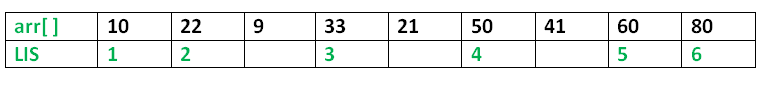Related Articles
Java Program for Longest Increasing Subsequence
• Difficulty Level : Medium
• Last Updated : 04 Dec, 2018

The Longest Increasing Subsequence (LIS) problem is to find the length of the longest subsequence of a given sequence such that all elements of the subsequence are sorted in increasing order. For example, the length of LIS for {10, 22, 9, 33, 21, 50, 41, 60, 80} is 6 and LIS is {10, 22, 33, 50, 60, 80}.More Examples:

```Input  : arr[] = {3, 10, 2, 1, 20}
Output : Length of LIS = 3
The longest increasing subsequence is 3, 10, 20

Input  : arr[] = {3, 2}
Output : Length of LIS = 1
The longest increasing subsequences are {3} and {2}

Input : arr[] = {50, 3, 10, 7, 40, 80}
Output : Length of LIS = 4
The longest increasing subsequence is {3, 7, 40, 80}
```

## Recommended: Please try your approach on {IDE} first, before moving on to the solution.

Optimal Substructure:
Let arr[0..n-1] be the input array and L(i) be the length of the LIS ending at index i such that arr[i] is the last element of the LIS.
Then, L(i) can be recursively written as:
L(i) = 1 + max( L(j) ) where 0 < j < i and arr[j] < arr[i]; or
L(i) = 1, if no such j exists.
To find the LIS for a given array, we need to return max(L(i)) where 0 < i < n.
Thus, we see the LIS problem satisfies the optimal substructure property as the main problem can be solved using solutions to subproblems.

Following is a simple recursive implementation of the LIS problem. It follows the recursive structure discussed above.

 `/* A Naive Java Program for LIS Implementation */` `class` `LIS { ` `    ``static` `int` `max_ref; ``// stores the LIS ` ` `  `    ``/* To make use of recursive calls, this function must return ` `   ``two things: ` `   ``1) Length of LIS ending with element arr[n-1]. We use ` `      ``max_ending_here for this purpose ` `   ``2) Overall maximum as the LIS may end with an element ` `      ``before arr[n-1] max_ref is used this purpose. ` `   ``The value of LIS of full array of size n is stored in ` `   ``*max_ref which is our final result */` `    ``static` `int` `_lis(``int` `arr[], ``int` `n) ` `    ``{ ` `        ``// base case ` `        ``if` `(n == ``1``) ` `            ``return` `1``; ` ` `  `        ``// 'max_ending_here' is length of LIS ending with arr[n-1] ` `        ``int` `res, max_ending_here = ``1``; ` ` `  `        ``/* Recursively get all LIS ending with arr, arr ... ` `           ``arr[n-2]. If   arr[i-1] is smaller than arr[n-1], and ` `           ``max ending with arr[n-1] needs to be updated, then ` `           ``update it */` `        ``for` `(``int` `i = ``1``; i < n; i++) { ` `            ``res = _lis(arr, i); ` `            ``if` `(arr[i - ``1``] < arr[n - ``1``] && res + ``1` `> max_ending_here) ` `                ``max_ending_here = res + ``1``; ` `        ``} ` ` `  `        ``// Compare max_ending_here with the overall max. And ` `        ``// update the overall max if needed ` `        ``if` `(max_ref < max_ending_here) ` `            ``max_ref = max_ending_here; ` ` `  `        ``// Return length of LIS ending with arr[n-1] ` `        ``return` `max_ending_here; ` `    ``} ` ` `  `    ``// The wrapper function for _lis() ` `    ``static` `int` `lis(``int` `arr[], ``int` `n) ` `    ``{ ` `        ``// The max variable holds the result ` `        ``max_ref = ``1``; ` ` `  `        ``// The function _lis() stores its result in max ` `        ``_lis(arr, n); ` ` `  `        ``// returns max ` `        ``return` `max_ref; ` `    ``} ` ` `  `    ``// driver program to test above functions ` `    ``public` `static` `void` `main(String args[]) ` `    ``{ ` `        ``int` `arr[] = { ``10``, ``22``, ``9``, ``33``, ``21``, ``50``, ``41``, ``60` `}; ` `        ``int` `n = arr.length; ` `        ``System.out.println(``"Length of lis is "` `                           ``+ lis(arr, n) + ``"\n"``); ` `    ``} ` `} ` `/*This code is contributed by Rajat Mishra*/`

Output:

```Length of lis is 5
```

Overlapping Subproblems:
Considering the above implementation, following is recursion tree for an array of size 4. lis(n) gives us the length of LIS for arr[].

```              lis(4)
/        |
lis(3)    lis(2)   lis(1)
/           /
lis(2) lis(1) lis(1)
/
lis(1)
```

We can see that there are many subproblems which are solved again and again. So this problem has Overlapping Substructure property and recomputation of same subproblems can be avoided by either using Memoization or Tabulation. Following is a tabluated implementation for the LIS problem.

 `/* Dynamic Programming Java implementation of LIS problem */` ` `  `class` `LIS { ` `    ``/* lis() returns the length of the longest increasing ` `       ``subsequence in arr[] of size n */` `    ``static` `int` `lis(``int` `arr[], ``int` `n) ` `    ``{ ` `        ``int` `lis[] = ``new` `int``[n]; ` `        ``int` `i, j, max = ``0``; ` ` `  `        ``/* Initialize LIS values for all indexes */` `        ``for` `(i = ``0``; i < n; i++) ` `            ``lis[i] = ``1``; ` ` `  `        ``/* Compute optimized LIS values in bottom up manner */` `        ``for` `(i = ``1``; i < n; i++) ` `            ``for` `(j = ``0``; j < i; j++) ` `                ``if` `(arr[i] > arr[j] && lis[i] < lis[j] + ``1``) ` `                    ``lis[i] = lis[j] + ``1``; ` ` `  `        ``/* Pick maximum of all LIS values */` `        ``for` `(i = ``0``; i < n; i++) ` `            ``if` `(max < lis[i]) ` `                ``max = lis[i]; ` ` `  `        ``return` `max; ` `    ``} ` ` `  `    ``public` `static` `void` `main(String args[]) ` `    ``{ ` `        ``int` `arr[] = { ``10``, ``22``, ``9``, ``33``, ``21``, ``50``, ``41``, ``60` `}; ` `        ``int` `n = arr.length; ` `        ``System.out.println(``"Length of lis is "` `+ lis(arr, n) + ``"\n"``); ` `    ``} ` `} ` `/*This code is contributed by Rajat Mishra*/`

Output:

```Length of lis is 5
```

Please refer complete article on Dynamic Programming | Set 3 (Longest Increasing Subsequence) for more details!

Attention reader! Don’t stop learning now. Get hold of all the important DSA concepts with the DSA Self Paced Course at a student-friendly price and become industry ready.

My Personal Notes arrow_drop_up
Recommended Articles
Page :